# Accessing elements of a Pandas Series

Pandas Series is a one-dimensional labeled array capable of holding data of any type (integer, string, float, python objects, etc.). Labels need not be unique but must be a hashable type. An element in the series can be accessed similarly to that in an ndarray. Elements of a series can be accessed in two ways –

• Accessing Element from Series with Position
• Accessing Element Using Label (index)In this article, we are using “`nba.csv`” file, to download the CSV, click here.

### Accessing Element from Series with Position

In order to access the series element refers to the index number. Use the index operator [ ] to access an element in a series. The index must be an integer.
In order to access multiple elements from a series, we use Slice operation. Slice operation is performed on Series with the use of the colon(:). To print elements from beginning to a range use `[:Index]`, to print elements from end-use` [:-Index]`, to print elements from specific Index till the end use `[Index:]`, to print elements within a range, use [Start Index:End Index] and to print whole Series with the use of slicing operation, use `[:]`. Further, to print the whole Series in reverse order, use` [::-1]`.

Code #1: Accessing a first element of series

 `# import pandas and numpy  ` `import` `pandas as pd ` `import` `numpy as np ` ` `  `# creating simple array ` `data ``=` `np.array([``'g'``, ``'e'``, ``'e'``, ``'k'``, ``'s'``, ``'f'``, ``'o'``, ``'r'``, ``'g'``, ``'e'``, ``'e'``, ``'k'``, ``'s'``]) ` `ser ``=` `pd.Series(data) ` `  `  `  `  `# retrieve the first element ` `print``(ser[``0``]) `

Output :

```g
```

Code #2: Accessing first 5 elements of Series

 `# import pandas and numpy  ` `import` `pandas as pd ` `import` `numpy as np ` ` `  `# creating simple array ` `data ``=` `np.array([``'g'``, ``'e'``, ``'e'``, ``'k'``, ``'s'``, ``'f'``, ``'o'``, ``'r'``, ``'g'``, ``'e'``, ``'e'``, ``'k'``, ``'s'``]) ` `ser ``=` `pd.Series(data) ` `  `  `  `  `# retrieve the first element ` `print``(ser[:``5``]) `

Output :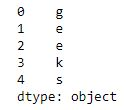Code #3: Accessing last 10 elements of Series

 `# import pandas and numpy  ` `import` `pandas as pd ` `import` `numpy as np ` ` `  `# creating simple array ` `data ``=` `np.array([``'g'``, ``'e'``, ``'e'``, ``'k'``, ``'s'``, ``'f'``, ``'o'``, ``'r'``, ``'g'``, ``'e'``, ``'e'``, ``'k'``, ``'s'``]) ` `ser ``=` `pd.Series(data) ` `  `  `  `  `# retrieve the first element ` `print``(ser[``-``10``:]) `

Output :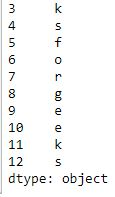Code #4: Accessing first 5 elements of Series in `nba.csv` file

 `# importing pandas module   ` `import` `pandas as pd   ` `     `  `# making data frame   ` `df ``=` `pd.read_csv(``"nba.csv"``)   ` `   `  `ser ``=` `pd.Series(df[``'Name'``])  ` `ser.head(``10``)  `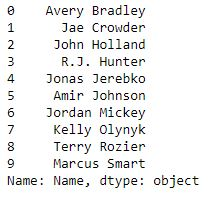Now we access first 5 elements of series

 `# get first five names  ` `ser[:``5``]  `

Output :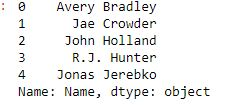### Accessing Element Using Label (index)

In order to access an element from series, we have to set values by index label. A Series is like a fixed-size dictionary in that you can get and set values by index label.

Code #1: Accessing a single element using index label

 `# import pandas and numpy  ` `import` `pandas as pd ` `import` `numpy as np ` ` `  `# creating simple array ` `data ``=` `np.array([``'g'``, ``'e'``, ``'e'``, ``'k'``, ``'s'``, ``'f'``, ``'o'``, ``'r'``, ``'g'``, ``'e'``, ``'e'``, ``'k'``, ``'s'``]) ` `ser ``=` `pd.Series(data, index ``=``[``10``, ``11``, ``12``, ``13``, ``14``, ``15``, ``16``, ``17``, ``18``, ``19``, ``20``, ``21``, ``22``]) ` `  `  `  `  `# accessing a element using index element ` `print``(ser[``16``]) `

Output :

```o
```

Code #2: Accessing a multiple element using index label

 `# import pandas and numpy  ` `import` `pandas as pd ` `import` `numpy as np ` ` `  `# creating simple array ` `data ``=` `np.array([``'g'``, ``'e'``, ``'e'``, ``'k'``, ``'s'``, ``'f'``, ``'o'``, ``'r'``, ``'g'``, ``'e'``, ``'e'``, ``'k'``, ``'s'``]) ` `ser ``=` `pd.Series(data, index ``=``[``10``, ``11``, ``12``, ``13``, ``14``, ``15``, ``16``, ``17``, ``18``, ``19``, ``20``, ``21``, ``22``]) ` `  `  `  `  `# accessing a multiple element using  ` `# index element ` `print``(ser[[``10``, ``11``, ``12``, ``13``, ``14``]]) `

Output :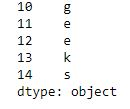Code #3: Access multiple elements by providing label of index

 `# importing pandas and numpy   ` `import` `pandas as pd   ` `import` `numpy as np  ` `   `  `ser ``=` `pd.Series(np.arange(``3``, ``9``), index ``=``[``'a'``, ``'b'``, ``'c'``, ``'d'``, ``'e'``, ``'f'``])  ` `   `  `print``(ser[[``'a'``, ``'d'``, ``'g'``, ``'l'``]]) `

Output :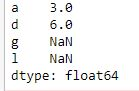Code #4: Accessing a multiple element using index label in `nba.csv` file

 `# importing pandas module   ` `import` `pandas as pd   ` `     `  `# making data frame   ` `df ``=` `pd.read_csv(``"nba.csv"``)   ` `   `  `ser ``=` `pd.Series(df[``'Name'``])  ` `ser.head(``10``)  `Now we access an multiple element using index label

 `ser[[``0``, ``3``, ``6``, ``9``]]  `

Output :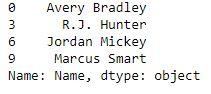My Personal Notes arrow_drop_upIf you like GeeksforGeeks and would like to contribute, you can also write an article using contribute.geeksforgeeks.org or mail your article to contribute@geeksforgeeks.org. See your article appearing on the GeeksforGeeks main page and help other Geeks.

Please Improve this article if you find anything incorrect by clicking on the "Improve Article" button below.

Article Tags :

2

Please write to us at contribute@geeksforgeeks.org to report any issue with the above content.